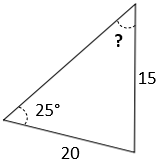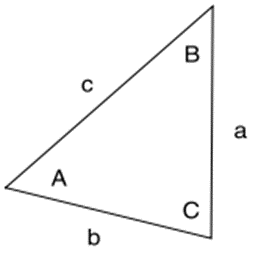## Law of Sines

For the triangle figure, what is the unknown angle in degrees?Hint
Law of Sines:
$$\frac{a}{sin\:A}=\frac{b}{sin\:B}=\frac{c}{sin\:C}$$$Hint 2For oblique (non-right) triangles, use the Law of Sines to determine unknown sides/angles: $$\frac{a}{sin\:A}=\frac{b}{sin\:B}=\frac{c}{sin\:C}$$$Thus, to find angle $$B$$ with the given knowns:
$$\frac{a}{sin\:A}=\frac{b}{sin\:B}$$$$$\frac{15}{sin\:25^{\circ}}=\frac{20}{sin\:B}$$$
$$sin\:B=\frac{20\cdot sin\:25^{\circ}}{15}=0.5635$$$$$B=sin^{-1}(0.5635)=34.3^{\circ}$$$
34.3°